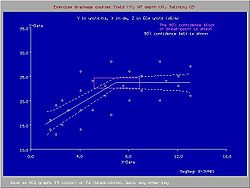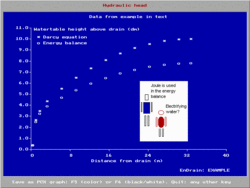# Drainage equation

﻿
Drainage equation

A drainage equation is an equation describing the relation between depth and spacing of parallel subsurface drains, depth of the watertable, depth and hydraulic conductivity of the soils. It is used in drainage design.Parameters in Hooghoudt's drainage equation

A well known steady-state drainage equation is the Hooghoudt drain spacing equation. Its original publication is in Dutch. The equation was introduced in the USA by van Schilfgaarde.

## Hooghoudt's equation

Hooghoudt's equation can be written as:.

Q L2 = 8 Kb d (Di - Dd) (Dd - Dw) + 4 Ka (Dd - Dw)2

where:

• Q = steady state drainage discharge rate (m/day)
• Ka = hydraulic conductivity of the soil above drain level (m/day)
• Kb = hydraulic conductivity of the soil below drain level (m/day)
• Di = depth of the impermeable layer below drain level (m)
• Dd = depth of the drains (m)
• Dw = steady state depth of the watertable midway between the drains (m)
• L = spacing between the drains (m)
• d = equivalent depth, a function of L, (Di-Dd), and r
• r = drain radius (m)

In steady state, the level of the water table remains constant and the discharge rate (Q) equals the rate of groundwater recharge (R), i.e. the amount of water entering the groundwater through the watertable per unit of time. By considering a long-term (e.g. seasonal) average depth of the water table (Dw) in combination with the long-term average recharge rate (R), the net storage of water in that period of time is negligibly small and the steady state condition is satisfied: one obtains a dynamic equilibrium.

Derivation of the equation
For the derivation of the equation Hooghoudt used the law of Darcy, the summation of circular potential functions and, for the determination of the influence of the impermeable layer, de method of mirror images and superposition.
Hooghoudt published tables for the determination of the equivalent depth (d), because the function (F) in d = F (L,Di-Dd,r) consists of long series of terms.Crop yield and seasonal average depth of the water table

Determining:

• the discharge rate (Q) from the recharge rate (R) in a water balance as detailed in the article: hydrology (agriculture)
• the permissible long term average depth of the water table (Dw) on the basis of agricultural drainage criteria
• the soil's hydraulic conductivity (Ka and Kb) by measurements
• the depth of the bottom of the aquifer (Di)

the design drain spacing (L) can be found from the equation in dependence of the drain depth (Dd) and drain radius (r).

Drainage criteria
One would not want the water table to be too shallow to avoid crop yield depression nor too deep to avoid drought conditions. This is a subject of drainage research. The figure shows that a seasonal average depth of the water table shallower than 70 cm causes a yield depression 
The figure was made with the SegReg program for segmented regression.

### Equivalent depth

In 1991 a closed-form expression was developed for the equivalent depth (d) that can replace the Hooghoudt tables  :

d = πL / 8 { ln(L/πr) + F(x) }

where:

• x = 2π (Di − Dd) / L
• F(x) = Σ 4e−2nx / n (1 − e−2nx) , with n = 1, 3, 5, . . .

### Extended use

Theoretically, Hooghoudt's equation can also be used for sloping land. The theory on drainage of sloping land is corroborated by the results of sand tank experiments. In addition, the entrance resistance encountered by the water upon entering the drains can be accounted for.

## AmplificationAmplification of parameters compared to Hooghoudt

The drainage formula can be amplified  to account for (see figure on the right):

• the additional energy associated with the incoming percolation water (recharge), see groundwater energy balance
• multiple soil layers
• anisotropric hydraulic conductivity, the vertical conductivity (Kv) being different from the horizontal (Kh)
• drains of different dimensions with any width (W)EnDrain: shape of the watertable with drainage

### Computer program

The amplified drainage equation uses an hydraulic equivalent of Joule's law in electricity.

It is in the form of a differential equation that cannot be solved analytically (i.e. in a closed form) but the solution requires a numerical method for which a computer program is indispensable.

The availability of a computer program also helps in quickly assessing various alternatives and performing a sensitivity analysis.

The blue figure shows an example of results of a computer aided calculation with the amplified drainage equation using the EnDrain program. It shows that incorporation of the incoming energy associated with the recharge leads to a somewhat deeper water table.

Wikimedia Foundation. 2010.

### Look at other dictionaries:

• Drainage research — is the study of agricultural drainage systems and their effects to arrive at optimal system design. Contents 1 Aspects to be covered 2 Systems analysis 3 Crop response processes 4 …   Wikipedia

• Drainage — See also: Storm drain and Stormwater Deep inside a Sydney drain in New South Wales Drainage is the natural or artificial removal of surface and sub surface water from an area. Many agricultural soils need drainage to improv …   Wikipedia

• Drainage system (agriculture) — An agricultural drainage system is a system by which the water level on or in the soil is controlled to enhance agricultural crop production. Contents 1 Classification 1.1 Surface drainage systems 1.2 Subsurface drainage systems …   Wikipedia

• Drainage model — may refer to: a surface drainage model or rainfall runoff model      see surface runoff, runoff model (reservoir) , or a software site providing free download of a conceptual rainfall runoff model (RainOff) based on a… …   Wikipedia

• Drainage Minier Acide — Pour les articles homonymes, voir DMA. Un drainage minier acide (DMA) ou encore « drainage rocheux acide » (DRA) est une solution minérale acide qui s écoule régulièrement en conséquence d une mine. Le phénomène en cause est spontané… …   Wikipédia en Français

• Drainage rocheux acide — Drainage minier acide Pour les articles homonymes, voir DMA. Un drainage minier acide (DMA) ou encore « drainage rocheux acide » (DRA) est une solution minérale acide qui s écoule régulièrement en conséquence d une mine. Le phénomène en …   Wikipédia en Français

• Drainage basin — Example of a drainage basin. The dashed line is the main water divide of the hydrographic basin A drainage basin is an extent or an area of land where surface water from rain and melting snow or ice converges to a single point, usually the exit… …   Wikipedia

• Drainage system (geomorphology) — In geomorphology, a drainage system is the pattern formed by the streams, rivers, and lakes in a particular drainage basin. They are governed by the topography of the land, whether a particular region is dominated by hard or soft rocks, and the… …   Wikipedia

• Drainage minier acide — Pour les articles homonymes, voir DMA. Exemple de drainage acide …   Wikipédia en Français

• Well drainage — means drainage of agricultural lands by wells. Agricultural land is drained by pumped wells (vertical drainage) to improve the soils by controlling water table levels and soil salinity. Contents 1 Introduction 2 Design 3 Flow to wells 4 …   Wikipedia